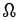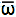# Parameters Describing Elliptical OrbitsSymbol Name Description Celestia EllipticalOrbit Parameter
. Apocenter The location of the greatest distance between the orbiting body and the central body when the orbit is an ellipse. The apocenter is diametrically opposite the pericenter on the major axis of the orbit. Not used
ω

lower case omega (ω), sometimes "w"
Argument of Pericenter The angle from the ascending node to the pericenter, measured in the plane of the orbit.
(Nobody knows why it's called "Argument". The earliest known use of the term "argument" to describe an angle was by Chaucer ~1391 in section 44 of his unfinished "A Treatise on the Astrolabe")
ArgOfPericenter
e
Eccentricity A measure of how elongated the orbit is.
• A circle has an eccentricity of 0.
• An ellipse has eccentricity between 0 and 1.
• A parabola has an eccentricity of 1.
• A hyperbola has an eccentricity greater than 1.
The eccentricity of an orbit can be calculated using one of several different formulae:
1. sqrt (1-(b^2/a^2))
where a is the semimajor axis and b is the semiminor axis
2. (apocenter - pericenter) / (apocenter + pericenter)
3. 1-(pericenter / semimajor axis)
Eccentricity
. Epoch A significant time, often the time at which the orbital elements for an object are valid. An epoch is usually specified as a Julian date. Epoch
i
Inclination The angle between this orbital plane and a reference plane. Inclination
. Line of Apsides The line between the Pericenter and the Apocenter. Not used
U
Line of Nodes The line between the Ascending Node and the Descending Node Not used
Ω
capital Omega, sometimes "W" orLongitude of the Ascending Node Angle from the origin of longitude of the reference plane to the orbit's ascending node: the point in its orbit where the orbiting body crosses the reference plane going "upward" or "northward". The time of this crossing is often used as the "elements' epoch." AscendingNodelower case omega (ω) with a bar over it (\bar ω)
Longitude of Pericenter Longitude of Ascending Node + Argument of Pericenter
This sometimes is called a "broken angle" since it includes angular measurements in two different planes.
LongOfPericenter
M
Mean Anomaly The location of the body in the orbit: the product of mean motion and time since pericenter passage. I.e., the mean anomaly is 0.0 when the orbiting body is at pericenter, so defining the orbital elements at the epoch Tp (the time of the pericenter passage) eliminates the need to determine the mean anomaly. MeanAnomaly
L
Mean Longitude Mean Longitude is the longitude that an orbiting body would have if its orbit were circular and its inclination were zero. It is equal to the true longitude only at pericenter and apocenter.

L = M += M + Ω + ω,
where

M is the orbit's Mean Anomaly,is the Longitude of Pericenter,

Ω is the Longitude of the Ascending Node and
ω is the Argument of Pericenter.
MeanLongitude
n (sometimes m)
Mean Motion Average angular velocity needed to complete one orbit
• For a closed orbit: e < 1.0
a = q/(1-e)
n = sqrt (G[M+m]/a^3) = elliptical mean motion
n = 1/P

• For a hyperbola: e > 1.0
which is not a closed orbit, one can use
a = q/(1-e)
n = sqrt (G[M+m]/[-a]^3) = hyperbolic mean motion.
(note that a is negative for hyperbolic orbits; hence the use of the minus sign to make the value of n positive.)

where
n is the Mean Motion
a is the length of the semimajor axis
q is the pericenter distance
e is the eccentricity of the orbit
G is the "gravitational constant"
M is the mass of the central object
m is the mass of the orbiting object
P is the period of the orbting object

Note: n is measured in Radians, not degrees.

Not used
q
Pericenter Distance The shortest distance between the center of the orbiting body and the center of the orbited body.
• q = a * (1 - e)
where q is the pericenter distance
a is the length of the semimajor axis and
e is the eccentricity of the orbit.
PericenterDistance
P
Period The time to complete one orbit.
• For a closed, elliptical solar orbit,
P = sqrt (( q / ( 1 - e)) ^3)
or = a^1.5

P = 1/n = 1/ Mean Motion

• For an open, hyperbolic orbit, one can use
P = sqrt (( q / ( e - 1)) ^3)

where
P is the period measured in years
q is the pericenter measured in AU
e is the eccentricity
and a is the semimajor axis of the orbit, also measured in AU (which has a negative value for hyperbolic orbits).

• More generally,
P = sqrt( K a^3 )
where a is the semimajor axis of the orbit,
and K is inversely proportional to the sum of the masses of the central body and the orbiting body.

When the mass of an orbiting body is small enough to ignore, the same value of K can be used for all small objects orbiting around the same central body. In other words, given the orbital period of one satellite, it's easy to calculate the periods of others. This is also known as "Kepler's Third Law."

Period
a
Semimajor Axis 1/2 of the length of the orbit's major axis; half of (the pericenter distance plus the apocenter distance)

a = ((m1+m2)*P^2)^(1/3)

where

a is the semimajor axis measured in AU,
m1 and m2 are the masses of the orbiting bodies measured in units of the mass of the Sun,
and P is the period of revolution about the barycenter measured in Years

SemiMajorAxis
Tp
Time of Pericenter Passage A time at which the orbiting body passes through the pericenter, closest to the central body. sometimes used as Epoch value

Notes:
• Orbital motion normally is counterclockwise when seen from above. (The "right handed" rule.)
• Angles are usually specified in degrees, although calculations often use radians. 2 π radians = 2*3.1459... radians = 360 degrees, 1 degree = .01745329 radians.
• Sometimes satellite orbits are described in terms of apocenter and pericenter (apogee and perigee) with no mention of eccentricity or period. Hence all four terms are included above along with conversion formulas.
• Sometimes satellite orbits are described misusing the terms apogee and perigee when what is really meant is the "Apogee Altitude" and "Perigee Altitude": the distance above the Earth's surface of apogee and perigee, assuming a mean equatorial radius of 6378.14 km (3443.92 nmi). (nmi = nautical miles)
• The orbital plane of a satellite orbiting the Earth precesses at a rate of about -10 x (R/a)^3.5 cos(i) degrees per day, where
R = Earth's mean equatorial radius,
a = altitude, and
i = inclination.
• Thanks to Grant Hutchison for clarifying the situation with regard to "mean motion" and hyperbolic orbits.